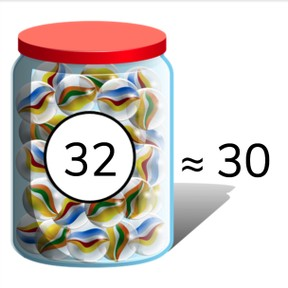Rounding to the nearest ten- numbers to 50

# Rounding to the nearest ten- numbers to 50

Rounding to the nearest ten- numbers to 508,000 schools use Gynzy92,000 teachers use Gynzy1,600,000 students use Gynzy

## General

Students learn how to determine the nearest tens number of numbers to 50. They do this using a number line. They learn to set the number line in their head and think about the number in the ones place to determine which tens number is closest to the given number.

## Relevance

It is important to be able to determine the nearest tens number to a given number, so you can calculate faster. You also need this to learn how to round numbers.

## Introduction

The interactive whiteboard shows a number line from 20 to 30. The students indicate which letter shows where 27 is on the number line. You can ask how they figure out this letter represents 27. Repeat this with the number 43 on a number line from 40 to 50.

## Development

Show the tens numbers on the board and discuss what tens numbers are with your students. Explain that a tens number has two digits, one in the ones place, and one in the tens place. The number in the tens place determines which tens a number is in. A tens number ends in a zero. An example of a tens number is 30. The number 34 is not a tens number because it has a four in the ones place. You can ask if students know any other tens numbers. The interactive whiteboard shows a number line with the numbers 30 and 40. Explain that you can see that 38 is closer to 40 than to 30. Discuss with students that the numbers 1,2,3, and 4 are closer to the tens below the given number, and the 6,7,8, and 9 that the number is closer to the tens above it.

To check that students can determine the nearest tens number of numbers to 50 you can ask the following questions and do the following exercises:
- How do you determine which tens number a given number is closest to?
- Is 38 closer to 30 or 40? How do you figure that out?
- Which tens number is 44 nearest to?

## Guided practice

Students determine if the given numbers are closer to one or another tens number using the visual support of a number line. Students are then asked to determine the nearest tens number without using a number line.

## Closing

Repeat the goal that they are able to round to the nearest tens number and ask why it is important for them to determine the nearest tens number. It is useful to be able to do this to speed up calculation, and useful if you want to know roughly how much or many of something you have. Ask the students how to determine if they go up to the next tens number or down to the previous tens number. The interactive whiteboard shows a few numbers between 40 and 50. Students must determine if the numbers are closer to 40 or 50. Ask which numbers indicate that a number goes down, namely 1,2,3 and 4; and which numbers show that it goes up, namely 6,7,8, and 9.

## Teaching tips

If students have difficulty rounding, they can use a number line for support on all exercises to count which tens number they are closest to. By counting steps the number that requires less steps is the nearest tens number.

### The online teaching platform for interactive whiteboards and displays in schools

• Save time building lessons

• Manage the classroom more efficiently

• Increase student engagement